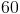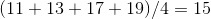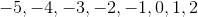# ACT Math : How to find the number of integers between two other integers

## Example Questions

### Example Question #1 : How To Find The Number Of Integers Between Two Other Integers

How many irrational numbers are there between 3 and 7?

8

10

4

Infinitely many

5

Infinitely many

Explanation:

There is an infinite amount of irrational numbers between any two "real" (whole) numbers. 3 and 7 are both real numbers

### Example Question #2 : How To Find The Number Of Integers Between Two Other Integers

3 candy bars and 4 bags of potato chips cost $7, and 3 candy bars and 2 bags of potato chips cost$5. What is the price of 1 bag of potato chips?

$.33$.50

$1$2

$1 Explanation: Increasing 2 bags of potato chips increases the cost by$2. Therefore, they cost \$1 per bag.

### Example Question #1 : How To Find The Number Of Integers Between Two Other Integers

What is the average of all prime numbers in between 7 and 23 (not including 7 or 23)?Explanation:

A prime number is defined as any number that is only divisible by itself and 1 with no remainders. So for example, 7 and 23 are both prime numbers. Seven can only be divided by 7 and 1, any other number would give a fraction or decimal.

The best approach to this problem is to simply list each number starting from 7.

Seven itself is a prime number, but the question specifically says that 7 should not be included. Eight is divisible by 1, 2, 4, and 8, and therefore is not a prime number. Nine is divisible by 3 and so on. Quickly we see that the prime numbers in betwen 7 and 23 include:Finally, the question asks you to find the average of these numbers:Concepts such as prime numbers or other definitions should be readily called when taking the ACT with minimal effort.

### Example Question #1 : How To Find The Number Of Integers Between Two Other Integers

How many prime numbers are between 20 and 50?

7

6

8

5

3

7

Explanation:

Prime numbers are numbers that cannot be divided evenly by any numbers besides itself and 1.  The prime numbers between 20 and 50 are: 23, 29, 31, 37, 41, 43, 47, yielding an answer of 6.

### Example Question #1 : How To Find The Number Of Integers Between Two Other Integers

How many integers are betweenand(not includingand)?The integers are.